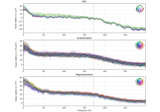# mne.time_frequency.psd_array_multitaper#

mne.time_frequency.psd_array_multitaper(x, sfreq, fmin=0.0, fmax=inf, bandwidth=None, adaptive=False, low_bias=True, normalization='length', output='power', n_jobs=None, *, max_iter=150, verbose=None)[source]#

Compute power spectral density (PSD) using a multi-taper method.

The power spectral density is computed with DPSS tapers.

Parameters:
x`array`, shape=(…, n_times)

The data to compute PSD from.

sfreq`float`

The sampling frequency.

fmin, fmax`float`

The lower- and upper-bound on frequencies of interest. Default is `fmin=0, fmax=np.inf` (spans all frequencies present in the data).

bandwidth`float`

Half-bandwidth of the multi-taper window function in Hz. For a given frequency, frequencies at ± half-bandwidth are smoothed together. The default value is a half-bandwidth of 4.

adaptive`bool`

Use adaptive weights to combine the tapered spectra into PSD (slow, use n_jobs >> 1 to speed up computation).

low_bias`bool`

Only use tapers with more than 90% spectral concentration within bandwidth.

normalization‘full’ | ‘length’

Normalization strategy. If “full”, the PSD will be normalized by the sampling rate as well as the length of the signal (as in Nitime). Default is `'length'`.

output`str`

The format of the returned `psds` array, `'complex'` or `'power'`:

• `'power'` : the power spectral density is returned.

• `'complex'` : the complex fourier coefficients are returned per taper.

n_jobs

The number of jobs to run in parallel. If `-1`, it is set to the number of CPU cores. Requires the `joblib` package. `None` (default) is a marker for ‘unset’ that will be interpreted as `n_jobs=1` (sequential execution) unless the call is performed under a `joblib.parallel_backend()` context manager that sets another value for `n_jobs`.

max_iter`int`

Maximum number of iterations to reach convergence when combining the tapered spectra with adaptive weights (see argument `adaptive`). This argument has not effect if `adaptive` is set to `False`.

verbose

Control verbosity of the logging output. If `None`, use the default verbosity level. See the logging documentation and `mne.verbose()` for details. Should only be passed as a keyword argument.

Returns:
psds`ndarray`, shape (…, n_freqs) or (…, n_tapers, n_freqs)

The power spectral densities. All dimensions up to the last (or the last two if `output='complex'`) will be the same as input.

freqs`array`

The frequency points in Hz of the PSD.

weights`ndarray`

The weights used for averaging across tapers. Only returned if `output='complex'`.

Notes

New in version 0.14.0.

References

## Examples using `mne.time_frequency.psd_array_multitaper`#The Spectrum and EpochsSpectrum classes: frequency-domain data

The Spectrum and EpochsSpectrum classes: frequency-domain data